Modelling and FE Simulation of HVC Using Multi Objective Response Surface Optimization Techniques

Modelling and FE Simulation of HVC Using Multi Objective Response Surface Optimization Techniques
Department of Mechanical Engineering, University of South Africa, Private Bag X6, Florida 1710, South Africa

Corresponding Author Email:
lmlab.unisa@gmail.com
Page:
307-315
|
DOI:
https://doi.org/10.18280/rcma.310601
2 October 2021
|
Accepted:
20 December 2021
|
Published:
31 December 2021
| Citation

OPEN ACCESS

Abstract:

The purpose of an automotive chassis is to maintain the shape of the vehicle and bear the various loads that are applied to the vehicle. The structure typically accounts for a large portion of the development and production costs of the new vehicle program, and the designer has many different structural concepts available. Choosing the best is important to ensure acceptable structural performance under other design constraints, such as cost, volume and method of production, product application, and more. The material selection for chassis depends upon various factors like lightness, economy, safety, recyclability, and circulation of life. The current study aims to perform optimization of the design of a heavy vehicle chassis using central composite design & optimal space fill design scheme (s) with the material tested is Al6092/SiC/17.5p MMC. Different design points are generated using design of experiments. The equivalent stress, deformation and mass are evaluated for each design points. The CAD modelling and FE simulation of heavy motor vehicle chassis is conducted using ANSYS software. From the optimization conducted on chassis design, response surface plots of equivalent stress, deformation and mass are generated which enabled to determine the range of dimensions for which these parameters are maximum or minimum. The use of Discontinuously Reinforced Aluminium-Matrix Composites Al6092/SiC/17.5p MMC aided to reduce weight of chassis by 66.25% and 66.68% by using CCD and Optimal space fill design scheme respectively, without much reduction in strength of chassis.

Keywords:

heavy vehicle chassis (HVC), automobile, stress, deformation, Al6092/SiC/17.5p MMC

1. Introduction

The findings presented here, are the part of a detailed research study. The aim of present paper is to perform a structural analysis of a heavy motor vehicle chassis & optimize it using different optimization methods to reduce the weight using Reinforced Aluminium-Matrix Composites Al6092/SiC/17.5p MMC. The appropriate data of an existing heavy duty truck chassis of TATA company’s model number 1612 (material used St52 E=2.10 x 105 N / mm2) as a simply supported beam with overhang ladder frame is taken for design and analysis with Side bar of the chassis made from ‘C’ Channels having dimensions 116mm x 25mm x 5 mm . Total load acting on the chassis is 257022N . The CAD modeling and FE simulation is conducted using ANSYS software.

A detailed static structural analysis is conducted in heavy motor vehicle chassis enabled to determine critical regions of high stresses and deformation. The suggestions are produced among the currently used conventional steel and suggested metal matrix composite (s). The variables selected for optimization are cross member width and scheme of optimization are central composite design & optimal space fill design of response surface methodology [18, 19].

2. Methodology

The geometric parameters of chassis such as lateral member dimensions have significant effect on stresses, weight and deformation generated on chassis structure which affects the strength of chassis. It is therefore necessary to evaluate the effect of these variables on output parameters (stress, deformation, and weight). The methodology involves Finite Element Analysis of chassis followed by design optimization using central composite design scheme followed by the optimal space filling design response surface method. The preprocessing stage in simulation involves CAD modeling, meshing, and applying loads and boundary conditions . The solution stage involves element matrix formulations, assemblage of global stiffness elements followed by inversions and multiplications . The final stage is post processing which involves viewing and editing of results where the chassis specifications were obtained from .

2.1 Simulation & modelling

The simulation form used in this study is static structural type. The version of 120 simulation package is ANSYS V18.1. The interaction to software is through graphical user 121 interface (GUI). However, the inbuilt language of software is C++. The CAD model of chassis is developed in ANSYS design modeler using extrude and sketch tool. Figure 1 shows the cross member 1, 2 and 3 in the developed CAD model.

1.pngFigure 1. Selected cross members in the CAD model

Subsequent process involves defining optimization variables i.e., cross member 1, cross member 2 and cross member 3. All the three dimensions selected for optimization are of linear type and each having length of 65mm shown in Figure 2.

2.pngFigure 2. Variable assignment

The chassis design has sharp edges and curvatures, and the tetrahedral element is suited for such complex geometries as shown in Figure 3 while Figure 4 shows the contact regions of the model.

3.pngFigure 3. Tetrahedral element

4.png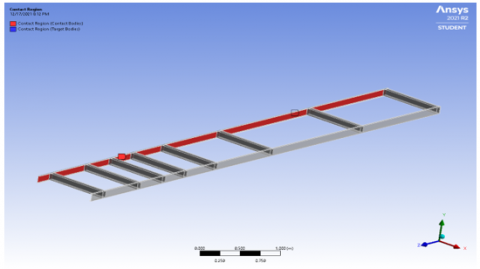Figure 4. Contact region of the model

The chassis design is discretized using tetrahedral elements with growth rate set to 1.2, inflation set to normal, and number of layers set to 5. The number of elements generated is 20080 and number of nodes generated is 42840. The next step involves applying loads and boundary conditions.

The boundary conditions are determined based on available literature  as well as analytical calculations which represents actual, physical conditions acting on HMV chassis. The FEA simulation conditions are same as experimental testing conditions. Total load acting on chassis = Capacity of the Chassis + Weight of body and engine= (25000+600+400+200) * 9.81 = 257022N load acting on each beam is half of the Load acting on the single frame= 257022 / 2 = 128511 N / Beam.

Chassis is simply clamp with Shock Absorber and Leaf Spring. So, Chassis is a Simply Supported Beam with uniformly distributed load and connections are welded type permanent joints. Load acting on Entire span of the beam is 128511N per beam.

Length of the Beam is 8810 mm. Uniformly Distributed Load is 128511/ 8810 =14.58 N/mm.

The downward direction force is applied on both longitudinal members and fixed support is applied on first and last transverse member as shown in Figure 5.

Conventionally used St52E is ductile material which possess isotropic behavior. Al6092/SiC/17.5p is an isotropic MMC with specific mechanical properties [22, 23].

The Al6092/SiC/17.5p has density 2.8 gm/cm3, Youngs Modulus (x) (GPa) 100, Thermal Conductivity 165 (W/m-K) (x-y) [24, 25]. The composite material is defined in material property cell of static structural module in ANSYS workbench.

The structural properties defined is modulus of elasticity, poisons ratio and density. The material properties are also indicated in the Table 1 below.

Table 1. Material property

 Material Density (gm/cm3) Youngs Modulus (GPa) Thermal Conductivity (W/m-k) St52E [17, 26] 7800 200 60.5 Al6092/SiC/17.5p [25, 27] 2.8 100 165

5.png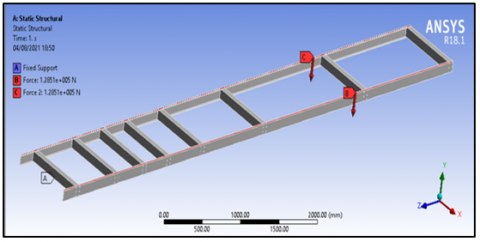Figure 5. Assigned loads and boundary conditions

After applying loads and boundary conditions, the solver is run using sparse matrix solver . The solution process involves generation of stiffness matrices associated with each element, assemblage of global stiffness matrix followed by matrix inversions and multiplication .

3. Result and Discussion

3.1 FE Results on a Standard Chassis using Al 6092/SiC/17.5P MMC

The FE simulation is conducted on chassis to determine stresses and deformation. The equivalent stress plot is shown in Figure 6. The equivalent stress observed for square section chassis is 3277.6MPa and 2446.4MPa is maximum stress on longitudinal member.

6.png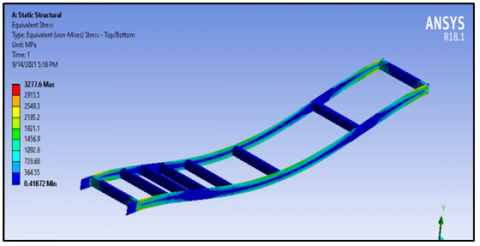Figure 6. Equivalent stress generated

The deformation obtained from the analysis is shown in Figure 7. The maximum deformation observed at the centre of chassis is 694.83 mm.

7.png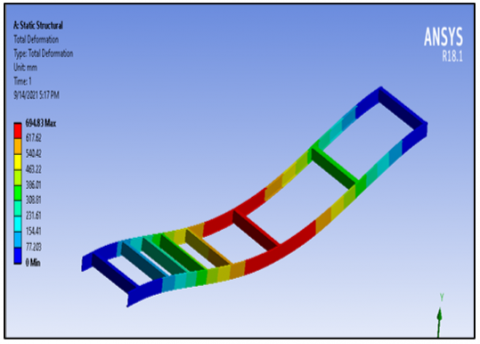Figure 7. Total deformation plot

3.2 Optimization of chassis using Al 6092/SiC/17.5P MMC

The stresses near the support are higher and is minimum at other zones. In order to improve the chassis’ response to such loads, the optimization is performed. The first optimization scheme used is the central composite design which has the three design points; axial, cube and center points. The software evaluated the output parameters at these design points using Finite Element Analysis. These output parameters are equivalent stress and the total deformation for CCD scheme are shown in column E and column F of the Table 2 (Appendix).

Second optimization scheme used is optimal space filling design (OSFD). The design points generated using optimal space filling design are shown in Table 3 (Appendix). Different combinations of cross member 1, cross member 2 and cross member 3 dimensions are generated based on optimal space filling design scheme.

The equivalent stress, deformation and solid mass are generated for each design point and the maximum and minimum values of these output parameters are shown in Table 4 (Appendix). The maximum equivalent stress obtained using optimal space filling design (OSFD) optimization is 3506.7MPa and minimum equivalent stress obtained from optimization is 3233MPa. The deformation and solid mass output show less variation between maximum and minimum values. The mass of chassis with structural steel is 214.64Kg whereas mass of chassis using Al/SiC MMC is 71.502Kg.

3.2.1 Deformation

Using CCD scheme, the maximum deformation is observed for design point number two (695.69 mm) and minimum deformation is observed for design point number seven (681.48 mm). The dimensions corresponding to the design point number two (2) is as follows:

• 58.5 mm for cross member 1 (-10%), 65 mm for cross member 2 and 65 mm for cross member 3.

The variation of total deformation vs design points is shown in Figure 8.

Using OSFD scheme, the maximum deformation is observed for design point number 12 (702.81 mm) and minimum deformation is observed for design point number ten (702.81mm) as shown in Figure 9. The dimensions corresponding to the design point number 12 is as follows:

• 60.667 mm for cross member 1 (-6.66%), 65 mm for cross member 2 and 61.533 mm (-5.33%) for cross member 3.

8.pngFigure 8. Total deformation vs design points for CCD scheme

9.pngFigure 9. Total deformation vs design points for OSFD scheme

The variation of solid mass vs design points for CCD scheme is shown in Figure 10.

10.png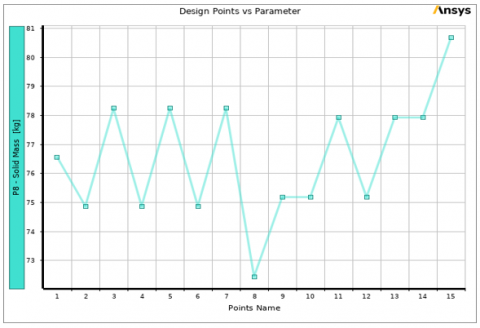Figure 10. Solid mass vs design points for CCD scheme

The maximum solid mass obtained from optimization is 80.673Kg as design point number 15 and minimum solid mass obtained from analysis is 72.447Kg at design point number 8.

3.2.2 Response surface analysis

The response surface plot aids to determine the range of values of optimization variables for which equivalent stress is maximum or minimum. The response surface plot of equivalent stress vs cross member 1 and cross member 2 using central composite design scheme is shown in Figure 11. Two peaks of equivalent stress are observed as shown in red colored region.

11.png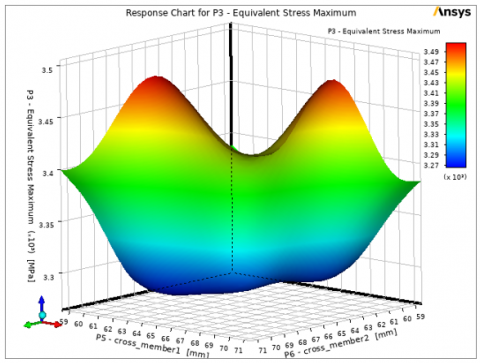Figure 11. Response surface plot of equivalent stress vs cross member 1 and cross member 2 for CCD scheme

As per the first peak appeared along cross member 1 dimension, the equivalent stress is maximum for cross member 1 dimension ranging from 62mm to 67mm and cross member 2 dimension ranging from 69mm to 71mm. As per the second peak appeared along cross member 2 dimension, the equivalent stress is maximum for cross member 1 dimension ranging from 69mm to 71mm and cross member 2 dimension ranging from 61mm to 67mm.

The equivalent stress is minimum for other dimensions of cross member 1 and cross member 2 as shown in dark blue colored region.

Figure 12 shows the response surface plot of equivalent stress vs cross member 1 and cross member 2 using OSFD scheme.

12.pngFigure 12. Response surface plot of equivalent stress vs cross member 1 and cross member 2 using OSFD

The response surface plot of equivalent stress shows 2 peaks as represented in red colored region. The maximum equivalent stress is obtained for cross member 2 ranging from 59mm to 61mm and cross member 1 ranging from 59mm to 61mm. The equivalent stress is observed to be minimum for regions represented in dark blue color.

The response surface plot of equivalent stress vs cross member 2 and cross member 3 using CCD scheme is shown in Figure 13.

13.pngFigure 13. Response surface plot of equivalent stress vs cross member 2 and cross member 3 for CCD Scheme

Single peak of equivalent stress is observed as shown in red colored region. The dimensions corresponding to maximum equivalent stress are obtained using interpolation method. The maximum equivalent stress is observed for cross member 3 value ranging from 61mm to 67mm and cross member 2 value ranging from 69mm to 71mm.

The equivalent stress is minimum for other values of cross member 2 and cross member which is represented by dark blue colored region.

The response surface plot of equivalent stress vs cross member 2 and cross member 3 using optimal space filling design is shown in Figure 14.

14.png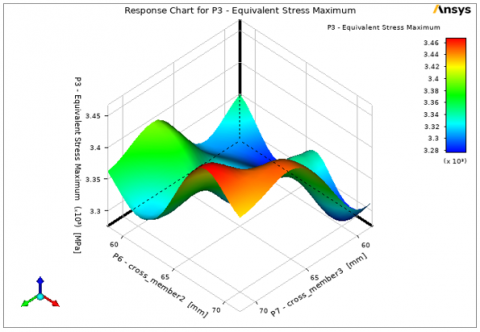Figure 14. Response surface plot of equivalent stress vs cross member 2 and cross member 3 using OSFD

The plot shows maximum equivalent stress in the region represented by red color for which the cross member 2-dimension ranges from 66mm to 71mm whereas cross member 3 ranges from 66mm to 71mm. The equivalent stress is minimum for region represented in dark blue color. The variation of equivalent stress with respect to cross member 1 for CCD scheme is shown in Figure 15. The graph shows gradual increase in equivalent stress up to 65mm.

The equivalent stress then increases exponentially and reaches maximum value of 3510Mpa at cross member dimension of 71mm while the variation of equivalent stress with respect to cross member 3 indicates the gradual increase in equivalent stress up to 63mm cross member 3 dimension and then decreases linearly with increases in cross member 3 dimension. The minimum equivalent stress is observed with cross member 3 dimension of 71.5mm.

15.png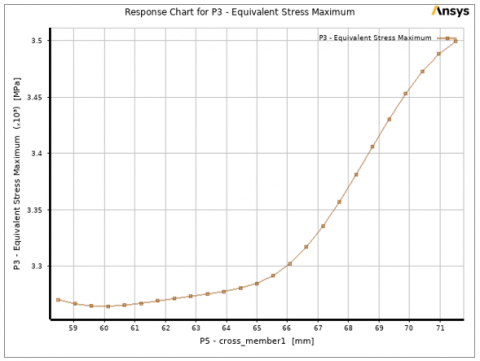Figure 15. Equivalent stress vs cross member 1 for CCD

The variation of equivalent stress vs cross member_1 using optimal space filling design is shown in Figure 16. The equivalent stress initially decreases and reaches minimum value at cross_member1 dimension of 66mm and then increases linearly and reaches maximum value at cross_member1 dimension of 71mm.

16.pngFigure 16. Equivalent stress vs cross_member 1 using optimal space filling design

The variation of equivalent stress vs cross_member3 indicates the equivalent stress increases and then decreases to reach minimum value at cross_member 3 value of 64mm. The equivalent stress then increases linearly to reach maximum value at cross member 3 dimension of 71mm. It is observed from the variation of equivalent stress vs cross member_2 that the equivalent stress initially decreases up to 64mm and then then increases steadily and reaches maximum value at 71mm and then decreases thereafter.

The variation of mass with respect to cross member 1 and cross member 2 for CCD scheme is shown in response surface plot of Figure 17. The maximum mass is represented in red colored region whereas the minimum mass is represented in blue colored region.

The maximum mass is observed for cross member 1 dimension ranging from 68mm to 71mm and cross member 2 dimension ranging from 68mm to 71mm. The minimum mass is observed for cross member 1 and cross member 3 dimensions ranging from 58.5mm to 61mm. The solid mass is observed to increase linearly with increase in cross member 1 dimension and cross member 3 dimensions. The minimum mass of chassis is observed for cross member 1 dimension of 58.5mm. The minimum mass of chassis is observed for 58.5mm cross member 3 and cross member 1 dimension.

17.png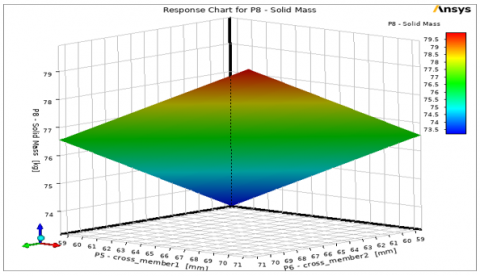Figure 17. 3D response surface plot of solid mass for CCD

The response surface plot of deformation cross member 1 and cross member 2 using optimal space filling design is shown in Figure 18. The plot shows maximum deformation for cross_member 2 dimension ranging from 59mm to 62mm and cross member 1 dimension ranging from 59mm to 67mm.

18.png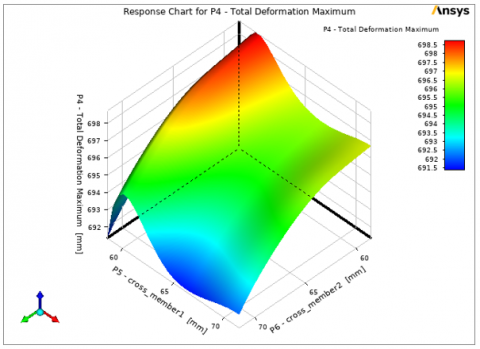Figure 18. Response surface plot of total deformation vs cross member 1 and cross member 2 using OSFD

For other dimensions the deformation shows minimum value as represented by blue colored zone. The variation of deformation vs cross member 2 and cross member 3 using optimal space filling design indicates the deformation to be maximum for cross member 3 dimension ranging from 61mm to 63mm and cross member 2 dimension ranging from 59mm to 71mm. The variation of mass vs cross member 1 and cross member 2 using optimal space filling design is shown in Figure 19.

The plot shows maximum solid mass for cross member 1 dimension ranging from 69mm to 71mm and cross member 2 dimension ranging from 69mm and 71mm. The mass is minimum for cross member 1 and cross member 2 dimension ranging from 59mm to 62mm. Figure 20 represents the sensitivity plot using CCD scheme. For equivalent stress, the maximum sensitivity percentage is shown by cross member 1 (76.94%) and minimum sensitivity percentage is shown by cross member 3 (19.034%) which signifies that cross member 1 has maximum effect on equivalent stress and cross member 3 has minimum effect on equivalent stress.

19.png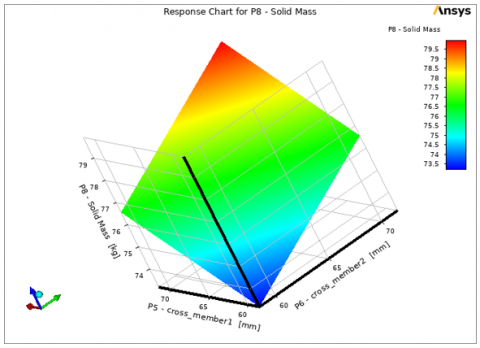Figure 19. Response surface plot of mass vs cross member 1 and cross member 2 using OSFD

20.png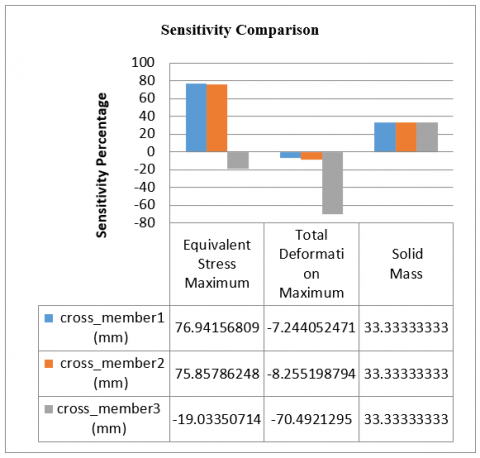Figure 20. Sensitivity plot using CCD scheme

For deformation, the maximum sensitivity percentage is shown by cross member 3 and minimum sensitivity percentage is shown by cross member 1. This signifies that cross member 3 has maximum effect on total deformation. For solid mass, all the three variables show the same sensitivity percentage which signifies that all the three optimization variables have the same effect on mass of chassis. The sensitivity plot using optimal space filling design is generated all the three output parameters i.e., equivalent stress, deformation, and solid mass in shown in Figure 21.

For equivalent stress, the cross member 1 shows maximum sensitivity percentage which signifies that cross member 2 has maximum effect on equivalent stress and cross member 3 has minimum effect on equivalent stress. For total deformation, the cross member 3 shows maximum sensitivity percentage which signifies that cross member 3 has maximum effect on total deformation. For solid mass, all the 3 optimization variables have same effect on mass of chassis.

21.pngFigure 21. Sensitivity plot using optimal space filling design

4. Conclusion

The new optimized design of chassis structure is presented using central composite design & optimal space fill optimization scheme. The response surface plots of different variables are generated which enabled to determine the range of values (of variables) for which equivalent stress deformation and mass is minimum or maximum. The weight of chassis is affected by width of cross members which is established by sensitivity plots. The minimum equivalent stress is observed with cross member 3 dimension of 71.5mm in CCD scheme and the equivalent stress increases linearly to reach maximum value at cross member 3 dimension of 71mm while using optimal space filling design. The mass of the chassis using the conventional steel is 214.64 Kg and it is reduced to 72.44 Kg (66.25%) in case of central composite design and 71.502 Kg (66.68%) in case of optimal space fill design optimization using Reinforced Aluminium-Matrix Composites Al6092/SiC/17.5p MMC. Therefore, optimal space fill design optimization is preferred in this case. The problem associated with conventional chassis materials is in achieving good combination of strength, stiffness, toughness, and density. These problems can be solved with the use of composite materials which are lighter and possess good strength.

Future Direction- The MMC’s can be a viable option for automotive components and the applicability of MMC for chassis structure are investigated in the current research using numerical techniques. The use of MMC’s for chassis structures aided to reduce the weight of chassis without compromise on its strength. In automotive industry, presently no work has been done in improving design of existing chassis using advanced optimization techniques & material which are used in this work. Further research can be conducted on chassis using other new materials & optimization techniques which could provide us better information on effect of other design variables.

Appendix

Table 2. DOE values for central composite design

 # Design Points of Design of Experiments # Central Composite Design # Name P5 - cross_member1 (mm) P6 - cross_member2 (mm) P7 - cross_member3 (mm) P3 - Equivalent Stress Maximum (MPa) P4 - Total Deformation Maximum (mm) P8 - Solid Mass (kg) 1.0000 65.0000 65.0000 65.0000 3277.6133 694.8263 76.5600 2.0000 58.5000 65.0000 65.0000 3261.5814 695.6932 74.8739 3.0000 71.5000 65.0000 65.0000 3514.8697 694.1797 78.2461 4.0000 65.0000 58.5000 65.0000 3270.7381 695.4341 74.8739 5.0000 65.0000 71.5000 65.0000 3522.2472 693.9100 78.2461 6.0000 65.0000 65.0000 58.5000 3256.6430 687.8316 74.8739 7.0000 65.0000 65.0000 71.5000 3217.4281 681.4766 78.2461 8.0000 59.7153 59.7153 59.7153 3344.0960 683.9605 72.4474 9.0000 70.2847 59.7153 59.7153 3429.5405 683.5024 75.1891 10.0000 59.7153 70.2847 59.7153 3435.5861 683.0817 75.1891 11.0000 70.2847 70.2847 59.7153 3312.0125 682.2530 77.9309 12.0000 59.7153 59.7153 70.2847 3461.2252 684.3118 75.1891 13.0000 70.2847 59.7153 70.2847 3228.5146 683.4313 77.9309 14.0000 59.7153 70.2847 70.2847 3291.4973 683.3310 77.9309 15.0000 70.2847 70.2847 70.2847 3458.8776 682.4539 80.6726

Table 3. DOE for optimal space-filling design scheme

 # Design Points of Design of Experiments: # Optimal Space-Filling Design # Name P5 - cross_member1 (mm) P6 - cross_member2 (mm) P7 - cross_member3 (mm) P3 - Equivalent Stress Maximum (MPa) P4 - Total Deformation Maximum (mm) P8 - Solid Mass (kg) 1.000 69.333 68.467 60.667 3489.332 690.154 77.459 2.000 63.267 69.333 59.800 3235.038 683.027 75.886 3.000 59.800 66.733 68.467 3351.487 688.864 76.560 4.000 68.467 59.800 67.600 3493.439 690.597 76.785 5.000 58.933 60.667 65.867 3501.736 694.025 74.087 6.000 71.067 63.267 63.267 3347.148 697.720 77.234 7.000 66.733 62.400 58.933 3246.612 685.046 74.761 8.000 70.200 65.867 69.333 3319.292 686.102 79.258 9.000 65.867 64.133 64.133 3283.425 696.444 76.335 10.000 65.000 67.600 71.067 3464.306 682.233 78.808 11.000 61.533 71.067 65.000 3474.065 694.224 77.234 12.000 60.667 65.000 61.533 3502.205 702.807 74.537 13.000 64.133 58.933 62.400 3308.882 700.824 74.087 14.000 67.600 70.200 66.733 3471.700 690.697 79.033 15.000 62.400 61.533 70.200 3233.554 685.076 76.335

Table 4. Max-min values for optimal space-filling design scheme

 A B C 1 Name Calculated Minimum Calculated Maximum 2 P3- - Equivalent Stress Maximum (MPa) 3207.3 3531.8 3 P4- Total Deformation Maximum (mm) 680.06 696.36 4 P8- Solid Mass (kg) 71.502 81.618
References

 Vijayan, S.N., Sendhilkumar, S., Kiran Babu, K.M. (2015). Design and analysis of automotive chassis considering cross design and analysis of automotive chassis considering cross-section and material. International Journal of Current Research, 7(5): 15697-15701.

 Cavazzuti, M., Splendi, L. (2011). Structural optimization of automotive chassis: theory, set up, design. Italy: Universita Degli Studi Di Modena E Reggio Emila.

 Chiandussi, G., Gaviglio, I., Ibba, A. (2004). Topology optimisation of an automotive component without final volume constraint specification. Advances in Engineering Software, 35(10-11): 609-617. https://doi.org/10.1016/J.Advengsoft.2003.07.002

 Pedersen, C. (2004). Crashworthiness design of transient frame structures using topology optimization. Computer Methods in Applied Mechanics and Engineering, 193(6-8): 653-678. https://doi.org/10.1016/j.cma.2003.11.001

 Letsatsi, M.T., Agarwal, A., Pitso, I. (2021). The finite element evaluation of support bracket using the application of topological optimization. Journal of Engineering Research, 1-13. https://doi.org/10.36909/Jer.Icippsd.15505

 Duddeck, F. (2008). Multidisciplinary optimization of car bodies. Structural and Multidisciplinary Optimization, 35(4): 375-389. https://doi.org/10.1007/S00158-007-0130-6

 Reddy, C.M.M. (2014). Modeling and analysis of container chassis using Fem. Iosr Journal of Engineering, 4(1): 34-37. https://doi.org/10.9790/3021-04153437

 Swami, K.I., Tuljapure, S.B. (2014). Analysis of ladder chassis of Eicher 20.16 using fem. Iosr Journal of Applied Geology and Geophysics, 2(1): 6-13. https://doi.org//10.9790/0990-02110613

 Godse, S., Patel, D. (2013). Static load analysis of tata ace ex chassis and stress optimisation using reinforcement technique. International Journal of Engineering Trends and Technology, 4(7): 55-58.

 Sharma, A., Kumar, P., Jabbar, A., Khan, M.M. (2014). Structural analysis of a heavy vehicle chassis made of different alloys by different cross sections. International Journal of Engineering Research & Technology (IJERT), 3(6): 1778-1785.

 Nalawade, K.G., Sabu, A., P, B. (2014). Dynamic (vibrational) and static structural analysis of ladder frame. International Journal of Engineering Trends and Technology, 11(2): 93-98. https://doi.org/10.14445/22315381/ijett-v11p218

 Singh, A., Soni, V., Singh, A. (2014). Structural analysis of ladder chassis for higher strength. International Journal of Emerging Technology and Advanced Engineering, 4(2): 254-259.

 Anurag, Singh, A., Tripathi, A., Tiwari, A., Upadhyay, N., Lal, S. (2016). Design and analysis of chassis frame. International Journal of Research and Engineering, 3(4): 31-34.

 Agarwal, A., Molwane, O.B., Letsatsi, M.T. (2021). Experimental investigation & analysis of heat transfer characteristics in automotive mmc disc brake under steady state and dynamic conditions. Journal of Engineering Research. https://doi.org/10.36909/jer.icippsd.15527

 Kainer, K.U. (2006). Metal Matrix Composites: Custom-Made Materials for Automotive and Aerospace Engineering. Wiley-vch verlag gmbh.

 Rohatgi, P.K., Xiang, C., Gupta, N. (2018). 4.11 aqueous corrosion of metal matrix composites. Comprehensive Composite Materials II, 4: 287-312. https://doi.org/10.1016/b978-0-12-803581-8.09985-9

 Agarwal, A., Mthembu, L. (2021). Numerical modelling and multi objective optimization analysis of heavy vehicle chassis. Processes, 9(11): 2028. https://doi.org/10.3390/pr9112028

 Plasun, R. (n.d.). Central composite designs. https://www.iue.tuwien.ac.at/phd/plasun/node32.html, accessed on Jan. 10, 2020.

 Overview of space-filling designs. (2018). https://www.datadvance.net/product/pseven-core/generic-tool-for-design-of-experiments/, accessed on Jan. 11, 2020.

 Agarwal, A., Molwane, O.B., Marumo, R. (2020). Design Optimization of Knuckle Stub Using Response Surface Optimization. In: Praveen Kumar A., Dirgantara T., Krishna P.V. (eds) Advances in Lightweight Materials and Structures. Springer Proceedings in Materials, vol 8. Springer, Singapore. https://doi.org/10.1007/978-981-15-7827-4_15

 Song, J.B., Song, R., Xiong, Z. (2018). Acoustic radiation features and structural-acoustic sensitivity of channel beam. Traitement du Signal, 35(1): 35-45. https://doi.org/10.3166/TS.35.35-45

 Tidke, N., Burande, D.H. (2017). Analysis of HCV chassis using FEA. International Engineering Research Journal, 1-5.

 De Oliveira, F.C.G., Borges, J.A.F. (2008). Design and optimization of a space frame chassis. SAE Technical Paper Series. https://doi.org/10.4271/2008-36-0253

 Rawal, S.P. (2001). Metal-matrix composites for space applications. JOM, 53(4): 14-17. https://doi.org/10.1007/s11837-001-0139-z

 Chennakesava Reddy, A. (2018). Metal matrix composites - their properties and applications. https://jntuhceh.ac.in/web/tutorials/faculty/1674_composites.pdf.

 Ansys library file. (n.d.). https://www.ansys.com/academic/, accessed on Aug 15, 2021.

 Wang, W., Xu, R.Q., Hao, Y.X., Wang, Q., Yu, L.L., Che, Q.Y., Cai, Q.Y., Wang, K.S., Ma, Z.Y. (2018). Corrosion fatigue behavior of friction stir processed interstitial free steel. Journal of Materials Science & Technology, 34(1): 148-156. https://doi.org/10.1016/j.jmst.2017.11.013

 Marumo, R., Molwane, O.B., Agarwal, A. (2020). Numerical Analysis of Rear Spoilers in Improving Vehicle Traction. In: Praveen Kumar A., Dirgantara T., Krishna P.V. (eds) Advances in Lightweight Materials and Structures. Springer Proceedings in Materials, vol 8. Springer, Singapore. https://doi.org/10.1007/978-981-15-7827-4_16

 Agarwal, A., Mthembu, L. (2021). Weight optimization of heavy-duty truck chassis by optimal space fill design using light weight Graphite Al GA 7-230 MMC. Materials Today: Proceedings. http://dx.doi.org/j.matpr.2021.11.053